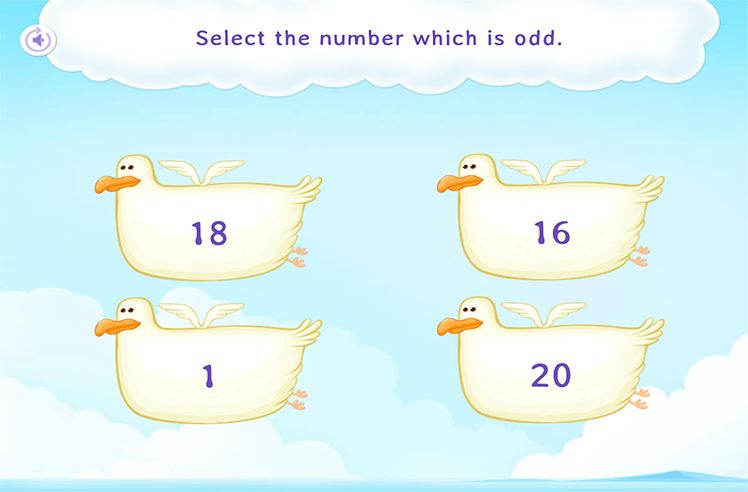# Descending Order – Definition with Examples

» Descending Order – Definition with Examples

## Descending Order

Arranging things, i.e., numbers, quantities, lengths, etc. from a larger value to lower value is known as descending order.  It is also known as the decreasing order.

For example,

Look at the numbers:

We can arrange these numbers in decreasing order of their value.

Descending can also be thought of as climbing down the stairs of numbers starting from the highest value.

Moving down the slide is descending.

The opposite of descending order is known as ascending order, in which the numbers are arranged from lower value to higher value.

## How to Finding Descending Order

The descending order of numbers can be found by subtracting 1 from the number.

For example, to write 10 to 6 in descending order, we will start with the largest number in the above sequence, which is 10 and keep subtracting 1 from it until we reach the lowest number.

So the numbers in descending order are:-

## Rules of Descending Order

Writing numbers in descending order do not always involve subtracting 1 from the larger numbers; rather, descending order can be done in the following manner:

Always ensure that the numbers are in order from largest to smallest

Always end with the smallest number

## Arranging the Measurement in Descending Order

We can use descending order to arranging the objects or people in decreasing order of their length or height.

For example, in the given image we show the height of animals in descending order.Number Sequence

Play NowNumber Recognition

Play NowEven and Odd Numbers

Play Now